Like   Tweet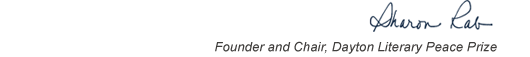table div table+table+table+table div table{width:100%;padding:0}table div table+table+table+table div table img{width:96.23%;padding:0;float:none}table div table+table+table+table div table td{width:100%;padding:0 1.88% 18px}/* styles */# Our Winners

 table div table+table+table+table+table+table div table{width:100%;padding:0}table div table+table+table+table+table+table div table img{width:96.23%;padding:0;float:none}table div table+table+table+table+table+table div table td{width:100%;padding:0 1.88% 18px}/* styles */# Prize in Fiction: The World We Knew by Alice Hoffman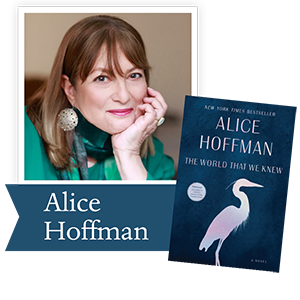Alice Hoffman is the author of more than thirty works of fiction, including Magic Lessons, The World That We Knew, Practical Magic, The Rules of Magic, the Oprah’s Book Club selection Here on Earth, The Red Garden, The Dovekeepers, The Museum of Extraordinary Things, The Marriage of Opposites, and Faithful. She lives near Boston.

 /* styles */ It is a great honor to be selected as the winner of the Dayton Peace Prize for my novel The World That We Knew, a book that explores what it means to be human in an inhuman time. Literature’s greatest gift is that it allows readers, and writers, to imagine ourselves living other lives, as other souls, in situations that challenge who we are and allow us to think about living a moral life. In writing about the Holocaust, especially now, at a time two thirds of millennials queried could not identify Auschwitz and 22 per cent had not heard of the Holocaust, this novel may be the most important work of my career. I want my readers to experience what it feels like is to be abandoned, ostracized, tortured, and murdered, as the result of being consider an outsider, just as I want them to feel what it is like to be loyal, to trust, to fight for justice, to love someone. It was my great privilege to meet with child survivors, now in their eighties and nineties, in this country and in France, and I was awed by their courage and humanity. Writing a novel that originated in their world was one of the great experiences of my life, one I will always be grateful for. —Alice Hoffman
 table div table+table+table+table+table+table+table+table+table+table div table{width:100%;padding:0}table div table+table+table+table+table+table+table+table+table+table div table img{width:96.23%;padding:0;float:none}table div table+table+table+table+table+table+table+table+table+table div table td{width:100%;padding:0 1.88% 18px}/* styles */# Prize in Nonfiction: Know My Name by Chanel MillerChanel Miller is a writer and artist who received her BA in Literature from the University of California, Santa Barbara. Her critically acclaimed memoir, Know My Name, was a New York Times bestseller, a New York Times Book Review Notable Book, and a National Book Critics Circle Award winner, as well as a best book of 2019 in Time, the Washington Post, the Chicago Tribune, NPR, and People, among others. She is a 2019 Time Next 100 honoree and a 2016 Glamour Woman of the Year honoree under her pseudonym, “Emily Doe.”

 /* styles */ In court, you testify in a wooden box and fear your words will be snuffed out at any moment. It’s that constant extinguishing that really wears you out. All that competing just to speak. Sitting down to write was the first time I could hear myself. Two o’clock in the morning, sitting in front of a blinking cursor on a blank screen in the quiet was the best thing that’s ever happened to me. No interruptions, nothing occurring, save for a small fan whirring. And then my book came out, solid as a brick, and I was still a nervous person. But one day I walked past a bookstore and saw my book, postured and proud and forward facing. And I understood that even if I slipped off the face of the earth tomorrow, my story would remain. I am in satchels and backpacks. I have fallen off of bedside tables, half tucked under the bed. My voice is indestructible. And there is a girl out there, who may be feeling as suffocated or hidden as I once was. Late at night, she’ll take out my book, and we’ll talk about the hardest parts, lay bare our buried feelings, and nobody can touch that space, and that to me is peace. —Chanel Miller
 table div table+table+table+table+table+table+table+table+table+table+table+table+table+table div table{width:100%;padding:0}table div table+table+table+table+table+table+table+table+table+table+table+table+table+table div table img{width:96.23%;padding:0;float:none}table div table+table+table+table+table+table+table+table+table+table+table+table+table+table div table td{width:100%;padding:0 1.88% 18px}/* styles */# Runner-Up in Fiction: The Beekeeper of Aleppo by Christy Lefteri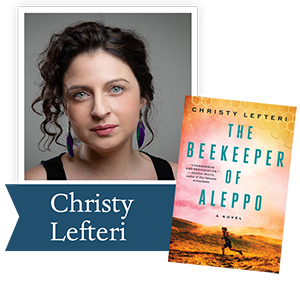Brought up in London, Christy Lefteri is the child of Cypriot refugees. She holds a PhD in creative writing from Brunel University where she is now a lecturer. Her previous novel, the international bestseller The Beekeeper of Aleppo was born out of her time working as a volunteer at a UNICEF-supported refugee center. She is also the author of A Watermelon, a Fish and a Bible, which was longlisted for the IMPAC Dublin Literary Award.

 /* styles */ Empathy is the beginning of peace. It is the seed from which peace grows. When we can say - I feel your pain, I might not know you but I will never add to your pain, - the possibility of peace comes into existence. Without empathy, peace is impossible; it is drowned in conflict, ideologies, prejudice, hatred, apathy. Without empathy, peace is dead. Powerful stories can cut through prejudices and bring us into the heart and mind of the other. Reading and hearing stories can help us to imagine lives that might otherwise be unimaginable. Stories can unite the self with the other, it can blur the lines and boundaries we make and force upon the world. A story can challenge our reactions to the thousands of images in the media, the streams of dehumanised people. It can awaken our emotions, make us focus on an individual so that they are no longer a face in a crowd. It can help us to imagine the feelings of fear and loss, devastation and trauma, love and hope and all the other emotions in between. A story can melt our hearts and our prejudices. If we can feel the pain of others and walk in their shoes, that’s a powerful starting place and my hope was that The Beekeeper of Aleppo would be able to achieve that. Empathy can move us to act when possible. It can help us to be mindful, and to take a step back, to give another space and the right to live happily and safely. Empathy is the starting point of peace and peace is a complex puzzle that needs to extend to the entire world and the entirety of living beings upon this world. So, when we can say I promise never to add to your pain and I will look after that promise as I look after my own life – then peace will blossom, one flower at a time. —Christy Lefteri
 table div table+table+table+table+table+table+table+table+table+table+table+table+table+table+table+table+table+table div table{width:100%;padding:0}table div table+table+table+table+table+table+table+table+table+table+table+table+table+table+table+table+table+table div table img{width:96.23%;padding:0;float:none}table div table+table+table+table+table+table+table+table+table+table+table+table+table+table+table+table+table+table div table td{width:100%;padding:0 1.88% 18px}/* styles */# Runner-Up in Nonfiction: Biased by Jennifer Eberhardt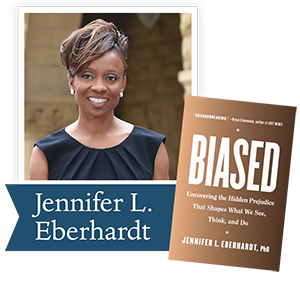Dr. Jennifer Eberhardt is a professor of psychology at Stanford and a recipient of a 2014 MacArthur “genius” grant. She has been elected to the National Academy of Sciences, the American Academy of Arts and Sciences, and was named one of Foreign Policy’s 100 Leading Global Thinkers. She is co-founder and co-director of SPARQ, a Stanford Center that brings together researchers and practitioners to address significant social problems.

 /* styles */ Bias does its work in the shadows and in the open, reworking our brains, framing and distorting our relationships with each other, erecting barriers that limit how we experience the world. Until we understand both its mechanics and its menace, we’re hostage to its power and cut off from the full measure of our own humanity. By sharing science, stories and history, the written word can dismantle our illusions and prompt the kind of soul searching that inspires hope and courage and fuels a thirst for justice, lighting the path to authentic peace. —Jennifer Eberhardt
 table div table+table+table+table+table+table+table+table+table+table+table+table+table+table+table+table+table+table+table+table+table+table div table{width:100%;padding:0}table div table+table+table+table+table+table+table+table+table+table+table+table+table+table+table+table+table+table+table+table+table+table div table img{width:96.23%;padding:0;float:none}table div table+table+table+table+table+table+table+table+table+table+table+table+table+table+table+table+table+table+table+table+table+table div table td{width:100%;padding:0 1.88% 18px}/* styles */table div table+table+table+table+table+table+table+table+table+table+table+table+table+table+table+table+table+table+table+table+table+table+table+table div table{width:100%;padding:0}table div table+table+table+table+table+table+table+table+table+table+table+table+table+table+table+table+table+table+table+table+table+table+table+table div table img{width:96.23%;padding:0;float:none}table div table+table+table+table+table+table+table+table+table+table+table+table+table+table+table+table+table+table+table+table+table+table+table+table div table td{width:100%;padding:0 1.88% 18px}/* styles */# UPCOMING EVENTS

 table div table+table+table+table+table+table+table+table+table+table+table+table+table+table+table+table+table+table+table+table+table+table+table+table+table+table div table{width:100%;padding:0}table div table+table+table+table+table+table+table+table+table+table+table+table+table+table+table+table+table+table+table+table+table+table+table+table+table+table div table img{width:96.23%;padding:0;float:none}table div table+table+table+table+table+table+table+table+table+table+table+table+table+table+table+table+table+table+table+table+table+table+table+table+table+table div table td{width:100%;padding:0 1.88% 18px}/* styles */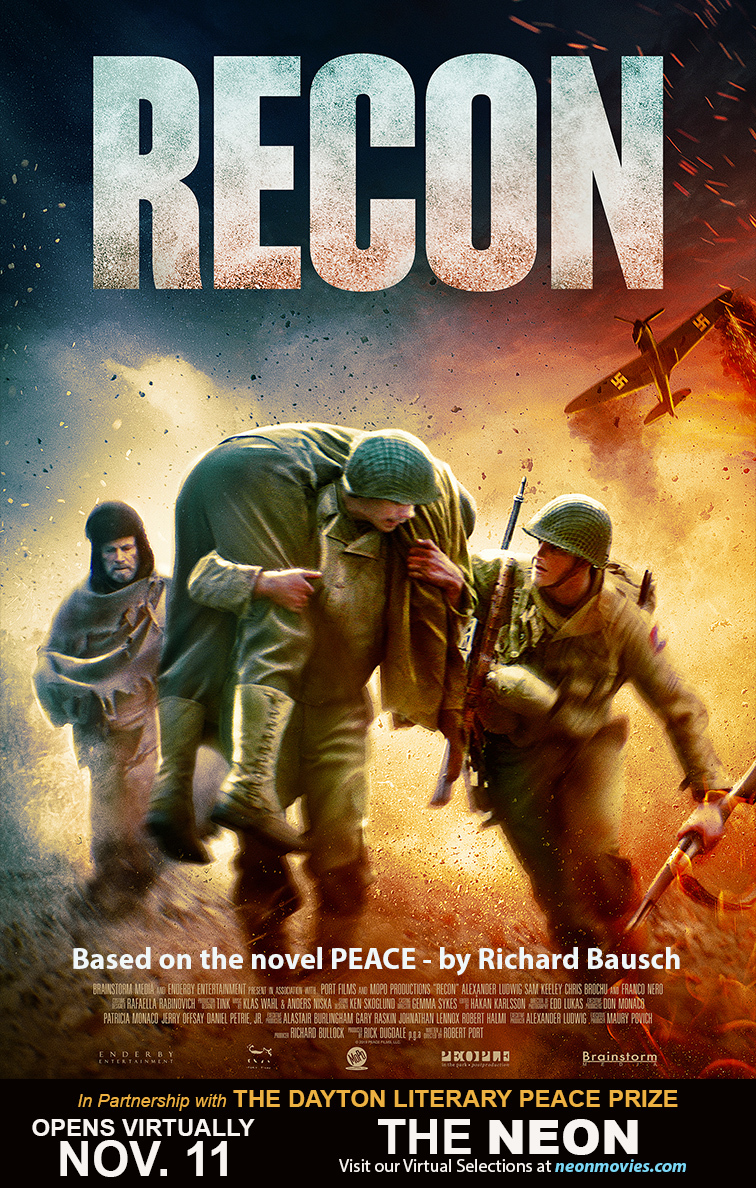# November 11: Opens Virtually

## Richard Bausch: RECON

Wednesday, November 11, 2020

Richard’s book, Peace, has been made into a film entitled RECON. It will debut in theaters on November 11 and will be streamed beginning November 13. Watch the trailer here.

Visit THE NEON's virtual selections.

 table div table+table+table+table+table+table+table+table+table+table+table+table+table+table+table+table+table+table+table+table+table+table+table+table+table+table+table+table div table{width:100%;padding:0}table div table+table+table+table+table+table+table+table+table+table+table+table+table+table+table+table+table+table+table+table+table+table+table+table+table+table+table+table div table img{width:96.23%;padding:0;float:none}table div table+table+table+table+table+table+table+table+table+table+table+table+table+table+table+table+table+table+table+table+table+table+table+table+table+table+table+table div table td{width:100%;padding:0 1.88% 18px}/* styles */# Turn the Page November 16

## The Legacy of War

 table div table+table+table+table+table+table+table+table+table+table+table+table+table+table+table+table+table+table+table+table+table+table+table+table+table+table+table+table+table+table div table{width:100%;padding:0}table div table+table+table+table+table+table+table+table+table+table+table+table+table+table+table+table+table+table+table+table+table+table+table+table+table+table+table+table+table+table div table img{width:96.23%;padding:0;float:none}table div table+table+table+table+table+table+table+table+table+table+table+table+table+table+table+table+table+table+table+table+table+table+table+table+table+table+table+table+table+table div table td{width:100%;padding:0 1.88% 18px}/* styles */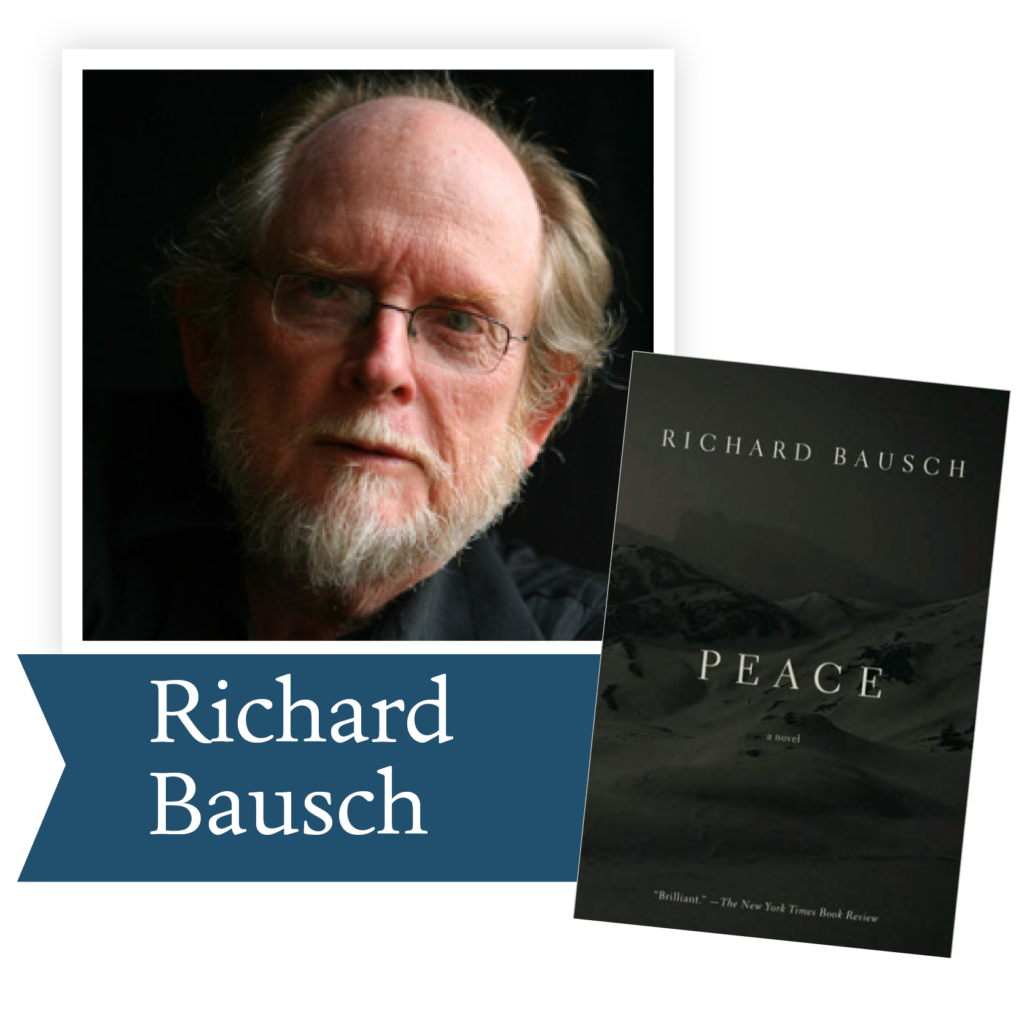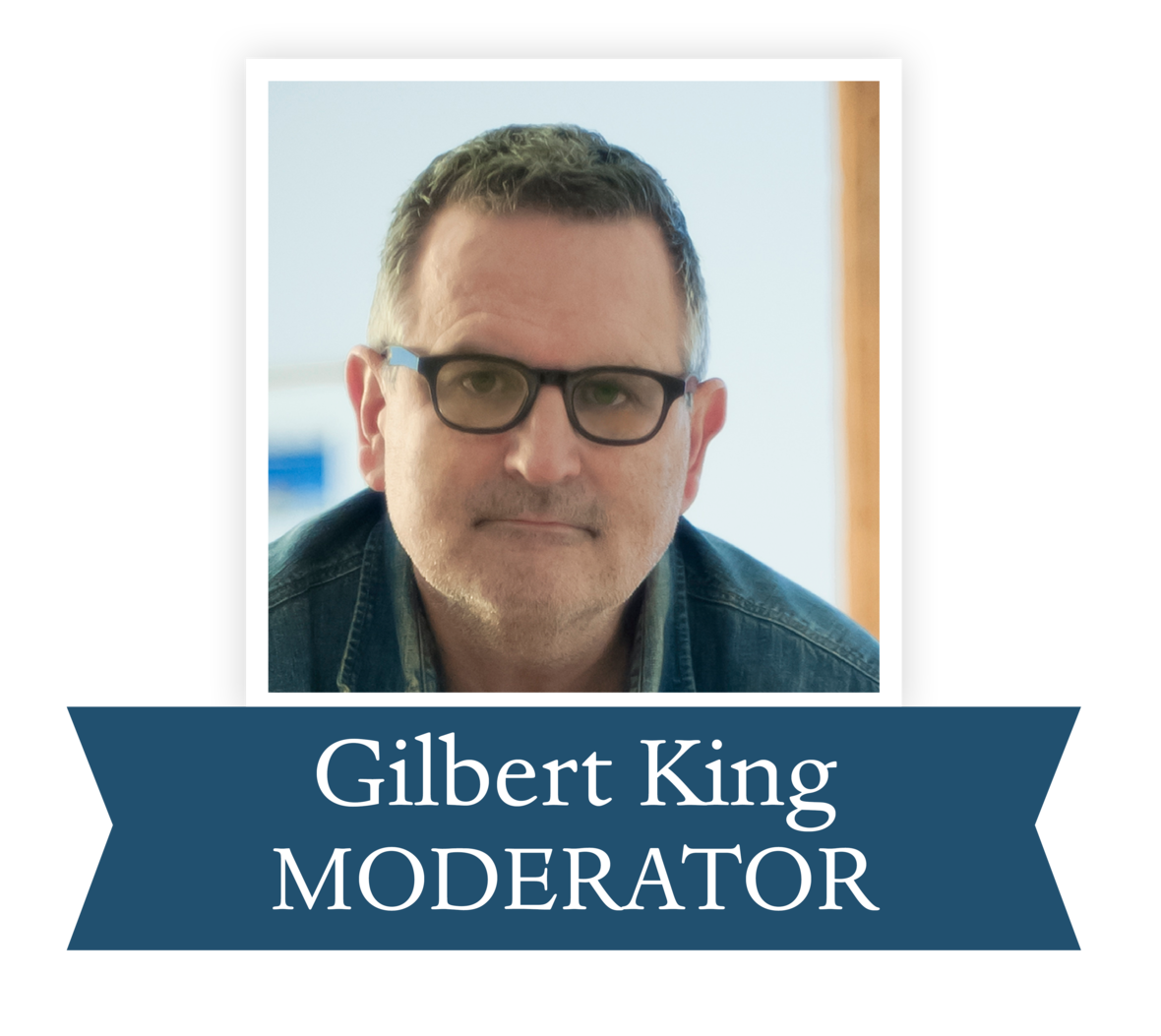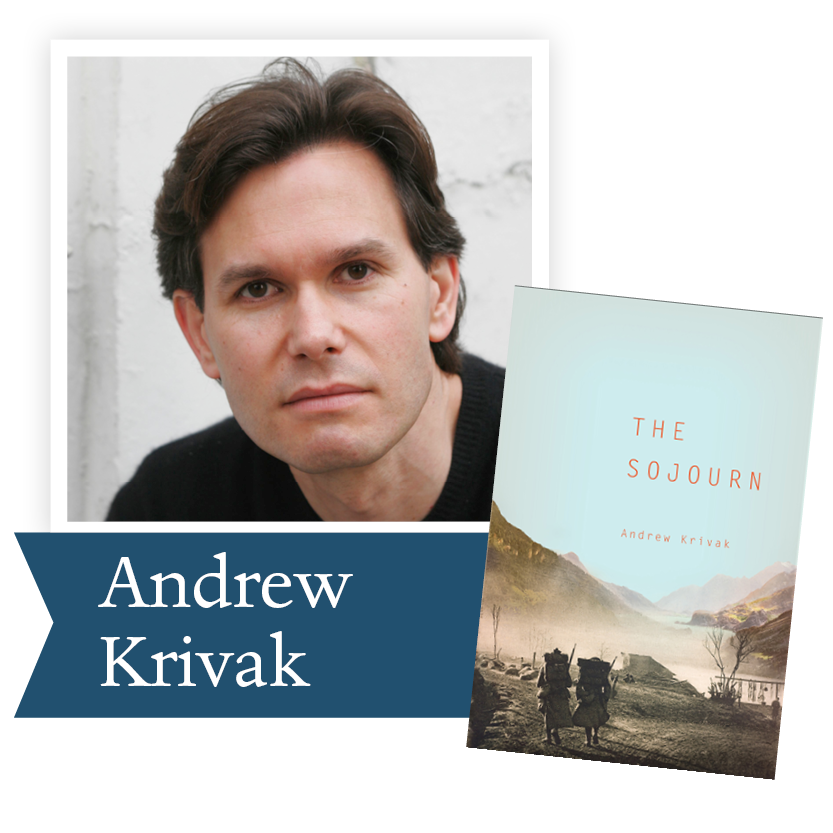/* styles */ More than a book club, Turn the Page becomes an agent for change, an evolution in thought brought about by a conversation with authors who have their fingers on the pulse of history, so therefore insight in the human heart. Each segment will bring two of our winning authors together to discuss a subject their works have in common. Gilbert King, a DLPP Honorary Advisory Board member, the 2013 DLPP Runner-up for The Devil in the Grove and the Pulitzer Prize winner for the same title, will moderate the sessions. The second Turn the Page event scheduled for November 16, at 7:00 EST will feature a conversation between Richard Bausch, the 2009 Fiction Winner for Peace, a novel set in the mountains of Italy with U.S. troops during World War II, and Andrew Krivak, the 2012 Fiction Winner for The Sojourn, set in those same mountains during World War I with a young man from Colorado who is a sharp shooter in the Kaiser’s Army. Both writers used family stories of war as the basis of their books and the springboards for their imagination. Richard’s book has been made into a film entitled RECON that will debut in theaters on November 11 and will be streamed beginning November 13. He will also discuss the experience of having his work translated to film. Register here to join us!
 table.module-32{width:47.92%;padding:0}table div table+table+table+table+table+table+table+table+table+table+table+table+table+table+table+table+table+table+table+table+table+table+table+table+table+table+table+table+table+table+table+table+table div table{width:47.92%;float:none;margin-left:auto;margin-right:auto;padding:0}table div table+table+table+table+table+table+table+table+table+table+table+table+table+table+table+table+table+table+table+table+table+table+table+table+table+table+table+table+table+table+table+table+table div table a{border:0 none;text-decoration:none}table div table+table+table+table+table+table+table+table+table+table+table+table+table+table+table+table+table+table+table+table+table+table+table+table+table+table+table+table+table+table+table+table+table div table img{width:100%!important;border:0 none;text-decoration:none}table div table+table+table+table+table+table+table+table+table+table+table+table+table+table+table+table+table+table+table+table+table+table+table+table+table+table+table+table+table+table+table+table+table div table td{width:100%;padding:0}/* styles */
 /* styles */ Watch the Turn the Page video here.
 table div table+table+table+table+table+table+table+table+table+table+table+table+table+table+table+table+table+table+table+table+table+table+table+table+table+table+table+table+table+table+table+table+table+table+table div table{width:100%;padding:0}table div table+table+table+table+table+table+table+table+table+table+table+table+table+table+table+table+table+table+table+table+table+table+table+table+table+table+table+table+table+table+table+table+table+table+table div table img{width:96.23%;padding:0;float:none}table div table+table+table+table+table+table+table+table+table+table+table+table+table+table+table+table+table+table+table+table+table+table+table+table+table+table+table+table+table+table+table+table+table+table+table div table td{width:100%;padding:0 1.88% 18px}/* styles */table div table+table+table+table+table+table+table+table+table+table+table+table+table+table+table+table+table+table+table+table+table+table+table+table+table+table+table+table+table+table+table+table+table+table+table+table+table div table{width:100%;padding:0}table div table+table+table+table+table+table+table+table+table+table+table+table+table+table+table+table+table+table+table+table+table+table+table+table+table+table+table+table+table+table+table+table+table+table+table+table+table div table img{width:96.23%;padding:0;float:none}table div table+table+table+table+table+table+table+table+table+table+table+table+table+table+table+table+table+table+table+table+table+table+table+table+table+table+table+table+table+table+table+table+table+table+table+table+table div table td{width:100%;padding:0 1.88% 18px}/* styles */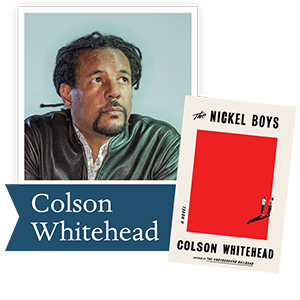Please join us for the next Dayton Literary Peace Prize Virtual Book Club on Wednesday, November 16, at 7:00 EST. Ron Rollins, recently retired editor of Ideas and Voices for the Dayton Daily News, is the host. November's featured book is The Nickel Boys by Colson Whitehead, one of the 2020 Finalists for the DLPP Fiction Award.

Mr. Colson has written seven books of fiction and two books of nonfiction over a 20-year career. He became only the second writer of color and sixth writer ever to win both a National Book Award and a Pulitzer Prize for the same novel, The Undergound Railroad.

The Nickel Boys, based on the notorious institution built in 1900 and originally known as the Florida State Reform School, follows two Black boys who suffer the beatings and abuses that had become the subject of damning reports for decades up until it closed in 2011, then known as the Dozier School for Boys. For more background, please read the Frank Rich review in the New York Times, In ‘The Nickel Boys,’ Colson Whitehead Depicts a Real-Life House of Horrors.

If you are interested in becoming a member of the book club, contact our Program Manager, Emily Kretzer.

 table div table+table+table+table+table+table+table+table+table+table+table+table+table+table+table+table+table+table+table+table+table+table+table+table+table+table+table+table+table+table+table+table+table+table+table+table+table+table+table div table{width:100%;padding:0}table div table+table+table+table+table+table+table+table+table+table+table+table+table+table+table+table+table+table+table+table+table+table+table+table+table+table+table+table+table+table+table+table+table+table+table+table+table+table+table div table img{width:96.23%;padding:0;float:none}table div table+table+table+table+table+table+table+table+table+table+table+table+table+table+table+table+table+table+table+table+table+table+table+table+table+table+table+table+table+table+table+table+table+table+table+table+table+table+table div table td{width:100%;padding:0 1.88% 18px}/* styles */# Support The Dayton Literary Peace Prize on Giving Tuesday

 table div table+table+table+table+table+table+table+table+table+table+table+table+table+table+table+table+table+table+table+table+table+table+table+table+table+table+table+table+table+table+table+table+table+table+table+table+table+table+table+table+table div table{width:100%;padding:0}table div table+table+table+table+table+table+table+table+table+table+table+table+table+table+table+table+table+table+table+table+table+table+table+table+table+table+table+table+table+table+table+table+table+table+table+table+table+table+table+table+table div table img{width:96.23%;padding:0;float:none}table div table+table+table+table+table+table+table+table+table+table+table+table+table+table+table+table+table+table+table+table+table+table+table+table+table+table+table+table+table+table+table+table+table+table+table+table+table+table+table+table+table div table td{width:100%;padding:0 1.88% 18px}/* styles */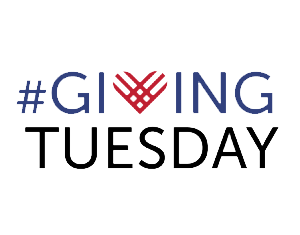Help the Dayton Literary Peace Prize reach our goal to spread peace through literature by providing 100 DLPP books to schools and libraries for #GivingTuesday

3 Ways You Can Get Involved on December 1st

1. Sponsor: For every \$25 donation to the Fly with the Doves Book Circle, you will provide one DLPP winning book to a school or library. Use this link to donate.
2. Share our Fly with the Doves page with family and friends.
3. Post an unselfie telling your friends why you support us (An unselfie is just like a selfie, but with a generous twist. Take a photo of yourself holding a sign that tells your followers who you’re supporting for #GivingTuesday and why.)
 1 Sponsor: For every \$25 donation to the Fly with the Doves Book Circle, you will provide one DLPP winning book to a school or library. Use this link to donate.
 2 Share our Fly with the Doves page with family and friends.
 3 Post an unselfie telling your friends why you support us (An unselfie is just like a selfie, but with a generous twist. Take a photo of yourself holding a sign that tells your followers who you’re supporting for #GivingTuesday and why.)
 table div table+table+table+table+table+table+table+table+table+table+table+table+table+table+table+table+table+table+table+table+table+table+table+table+table+table+table+table+table+table+table+table+table+table+table+table+table+table+table+table+table+table+table div table{width:100%;padding:0}table div table+table+table+table+table+table+table+table+table+table+table+table+table+table+table+table+table+table+table+table+table+table+table+table+table+table+table+table+table+table+table+table+table+table+table+table+table+table+table+table+table+table+table div table img{width:96.23%;padding:0;float:none}table div table+table+table+table+table+table+table+table+table+table+table+table+table+table+table+table+table+table+table+table+table+table+table+table+table+table+table+table+table+table+table+table+table+table+table+table+table+table+table+table+table+table+table div table td{width:100%;padding:0 1.88% 18px}/* styles */## Our 15th annual awards gala will take place June 27, 2021.

 table div table+table+table+table+table+table+table+table+table+table+table+table+table+table+table+table+table+table+table+table+table+table+table+table+table+table+table+table+table+table+table+table+table+table+table+table+table+table+table+table+table+table+table+table+table div table{width:100%;padding:0}table div table+table+table+table+table+table+table+table+table+table+table+table+table+table+table+table+table+table+table+table+table+table+table+table+table+table+table+table+table+table+table+table+table+table+table+table+table+table+table+table+table+table+table+table+table div table img{width:96.23%;padding:0;float:none}table div table+table+table+table+table+table+table+table+table+table+table+table+table+table+table+table+table+table+table+table+table+table+table+table+table+table+table+table+table+table+table+table+table+table+table+table+table+table+table+table+table+table+table+table+table div table td{width:100%;padding:0 1.88% 18px}/* styles */table div table+table+table+table+table+table+table+table+table+table+table+table+table+table+table+table+table+table+table+table+table+table+table+table+table+table+table+table+table+table+table+table+table+table+table+table+table+table+table+table+table+table+table+table+table+table div table,table.module-45{width:50.94%;float:left;padding:0}table div table+table+table+table+table+table+table+table+table+table+table+table+table+table+table+table+table+table+table+table+table+table+table+table+table+table+table+table+table+table+table+table+table+table+table+table+table+table+table+table+table+table+table+table+table+table div table a{border:0 none;text-decoration:none}table div table+table+table+table+table+table+table+table+table+table+table+table+table+table+table+table+table+table+table+table+table+table+table+table+table+table+table+table+table+table+table+table+table+table+table+table+table+table+table+table+table+table+table+table+table+table div table img{width:100%!important;border:0 none;text-decoration:none}table div table+table+table+table+table+table+table+table+table+table+table+table+table+table+table+table+table+table+table+table+table+table+table+table+table+table+table+table+table+table+table+table+table+table+table+table+table+table+table+table+table+table+table+table+table+table div table td{width:100%;padding:0 20px 20px 0}/* styles */ Support the Dayton Literary Peace Prize when you shop with Amazon using this link.